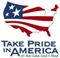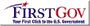Scientific Investigations Report 2006–5099

U.S. GEOLOGICAL SURVEY
Scientific Investigations Report 2006–5099

## Conversion Factors and Datums

### Inch/Pound to SI

Multiply By To obtain
cubic foot per second (ft3/s) 0.02832 cubic meter per second (m3/s)
cubic foot per second per mile [(ft3/s)/mi] 0.01760 cubic meter per second per kilometer [(m3/s)/km]
cubic foot per second per square mile [(ft3/s)/mi2] 0.01093 cubic meter per second per square kilometer [(m3/s)/km2]
foot (ft) 0.3048 meter (m)
foot per second (ft/s) 0.3048 meter per second (m/s)
foot per mile (ft/mi) 0.1894 meter per kilometer (m/km)
inch (in.) 25.4 millimeter (mm)
mile (mi) 1.609 kilometer (km)
square mile (mi2) 2.590 square kilometer (km2)

Temperature in degrees Celsius (°C) may be converted to degrees Fahrenheit (°F) as follows:

°F=(1.8 × °C)+32.

Temperature in degrees Fahrenheit (°F ) may be converted to degrees Celsius (°C) as follows:

°C = 0.556(°F-32).

Specific conductance is given in microsiemens per centimeter at 25 degrees Celsius (µS/cm at 25°C).

Instantaneous discharge is the discharge at a particular instant of time.

### Datums

Vertical coordinate information is referenced to the North American Vertical Datum of 1988 (NAVD 88). Horizontal coordinate information is referenced to the North American Datum of 1983 (NAD83).

Altitude, as used in this report, refers to distance above the vertical datum.U.S. Department of the Interior | U.S. Geological Survey